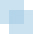# Present Value Models

##PRESENT VALUE OF ANY STREAM OF CASH FLOWS1

The present value of any stream of cash flows can be calculated by using Equation [A-1] or [A-2].where:

 PV = Present value= Sum of the periodic cash flows out to year n in increments i n = The last period for which cash flow is expected Ei = Expected cash flow in the ith period in the future k = Discount rate (usually the company's weighted average cost of capital adjusted for risk as appropriate) i = The period (usually in years) in the future in which the prospective cash flow is expected to be received

This series of cash flows is depicted in Figure A-1.FIGURE A-1 Stream of Cash Flows

Equation [A-2] is the expanded form of Equation [A-1] and can be used in a wide variety of applications. However, since this is a book about value, it's important for the reader to understand the context in which they will be used. In this appendix the objective is to further develop and simplify the present value equations depicted by [A-1] and [A-2] so they can be used to estimate the value of cash flows ...

Get Corporate Value Creation: An Operations Framework for Nonfinancial Managers now with the O’Reilly learning platform.

O’Reilly members experience books, live events, courses curated by job role, and more from O’Reilly and nearly 200 top publishers.